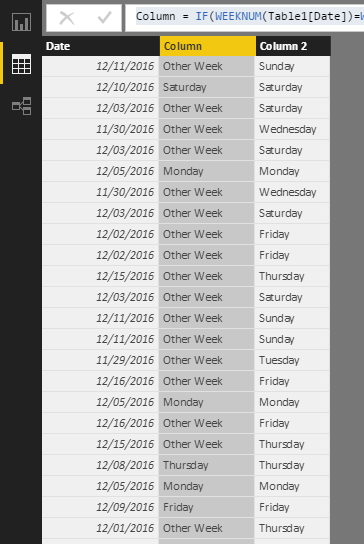cancel
Showing results for
Did you mean:Regular Visitor

## 2 DAX Questions

Hi, I am trying to figure out where I am going wrong with the below DAX Expression

=IF(AND(YEAR([Demand Sent]=YEAR(TODAY())),WEEKNUM([Demand Sent]=WEEKNUM(TODAY())),FORMAT([Demand Sent],"ddd"),""))

[Demand Sent] is a field that is either a date, or is blank. I am trying to get the dax expression to return "Mon" or "Tue" or "Wed" etc. if the date in this field is from the current week (Monday - Sunday) or return blank if its not.  the last peice, FORMAT([Demand Sent],"ddd"),""))  is giving me problems.

The second question is how can I use something comparable to vlookup in dax? I currently am using a vlookup to return a formula to then be applied to the current column.. this works in excel but not sure how to do it, or if its possible, to do this in dax..

=TODAY()-OFFSET(\$A22,0,VLOOKUP(\$AT22,BucketIDTable!G:I,3,FALSE)-1,1,1)

Any help with either question would be greatly appreciated!

1 ACCEPTED SOLUTIONMicrosoft

Hi @BGuttmann,

If you need check the year, you can use YEAR function.
Column = IF(WEEKNUM(Table1[Date])=WEEKNUM(TODAY())&&YEAR(Table1[Date])=YEAR(TODAY()),,SWITCH(WEEKDAY(Table1[Date]),1,"Sunday",2,"Monday",3,"Tuesday",4,"Wednesday",5,"Thursday",6,"Friday",7,"Saturday"),"Other Week")

And the week is start from Sunday.

Regards,

Charlie Liao

9 REPLIES 9Power Pivot is very different to Excel, and it is very common for Excel Pros to take an approach like this. But I am almost certain there is a better way to do what you want. It looks like you only have a single table. If you want to know day names, the. You should load a calendar table, create a relationship, and use the day name from the calendar table. http://exceleratorbi.com.au/power-pivot-calendar-tables/

Your VLOOKUP question is also probably the wrong question.  Can you describe your data and what you want to achieve?

* Matt is a Microsoft MVP (Power BI) and author of the Power BI Book Supercharge Power BI.Regular Visitor

Okay - i saw how to add the Calendar table and went ahead and did so. Can you tell me what the formula would be to check if a column date is from the current week and if so, return monday, tues,wed, thur,fri,sat or sun?Microsoft

Hi @BGuttmann,

To return weekday name if the date belong to current week, you can use the DAX below.
Column = IF(WEEKNUM(Table1[Date])=WEEKNUM(TODAY()),SWITCH(WEEKDAY(Table1[Date]),1,"Sunday",2,"Monday",3,"Tuesday",4,"Wednesday",5,"Thursday",6,"Friday",7,"Saturday"),"Other Week")Regards,

Charlie LiaoRegular Visitor

Hi - I just noticed that I dont think this is checking to make sure that the date is also from the current year - only the current week - how can I add that in? Also, is the "Current Week" being calculated for Monday - Sunday?Microsoft

Hi @BGuttmann,

If you need check the year, you can use YEAR function.
Column = IF(WEEKNUM(Table1[Date])=WEEKNUM(TODAY())&&YEAR(Table1[Date])=YEAR(TODAY()),,SWITCH(WEEKDAY(Table1[Date]),1,"Sunday",2,"Monday",3,"Tuesday",4,"Wednesday",5,"Thursday",6,"Friday",7,"Saturday"),"Other Week")

And the week is start from Sunday.

Regards,

Charlie LiaoRegular Visitor

Also, so how is this linking to the Calendar Table? Can I shorten the weekday names to 3 letters? (i.e. mon, tue,wed,thur)Regular Visitor

Okay, great - and one last question while I have you, I am using the below to form a unique string of numbers to identify what group a  particular file should fall into..

=CALCULATE(COUNT([Media Received])+0&COUNT([Demand Generated])+0&COUNT([Demand Atty Review])+0&COUNT([Demand Atty Approved])+0&COUNT([Demand Rejected])+0)&COUNT([Demand Sent])+0&COUNT([Demand Returned Date])+0&COUNT([Client Affidavit Requested Date])+0&COUNT([Client Affidavit Received])+0&COUNT([FDCPA Val Req Rec])+0&COUNT([Validation Letter Generated])+0&COUNT([Validation Reviewed By Atty])+0&COUNT([Validation Rejected])+0&COUNT([Validation Approved])+0&COUNT([Validation Sent])+0&COUNT([Complaint Generated Date])+0&COUNT([Complaint Reviewed By Attorney])+0&COUNT([Complaint Approved])+0)&CONCATENATE([DS<40 Days],[DR>DS]))

this is giving me an error though - where am I going wrong? I just want it to be a 1 if the field has a date and 0 if not..Regular Visitor

Sorry - guess I may have answered my own question here... would this be correct for the string of 1's and 0's or is there a better way to do it?

=CALCULATE(COUNT([Media Received])+0&CALCULATE(COUNT([Demand Generated])+0&CALCULATE(COUNT([Demand Atty Review])+0&CALCULATE(COUNT([Demand Atty Approved])+0&CALCULATE(COUNT([Demand Rejected])+0)&CALCULATE(COUNT([Demand Sent])+0&CALCULATE(COUNT([Demand Returned Date])+0&CALCULATE(COUNT([Client Affidavit Requested Date])+0&CALCULATE(COUNT([Client Affidavit Received])+0&CALCULATE(COUNT([FDCPA Val Req Rec])+0&CALCULATE(COUNT([Validation Letter Generated])+0&CALCULATE(COUNT([Validation Reviewed By Atty])+0&CALCULATE(COUNT([Validation Rejected])+0&CALCULATE(COUNT([Validation Approved])+0&CALCULATE(COUNT([Validation Sent])+0&CALCULATE(COUNT([Complaint Generated Date])+0&CALCULATE(COUNT([Complaint Reviewed By Attorney])+0&CALCULATE(COUNT([Complaint Approved])+0)))))))))))))))))&CONCATENATE([DS<40 Days],[DR>DS])Regular Visitor

I see - okay, so is the "calendar table" just adding a column? I dont see how to add a new table without importing data to powerpivot.

as to the vlookup -- what i am trying to do is take value (what I am calling a bucket) and then look that up in a vlookup.. which then tells me which column to subtract today() from.. basically, I want to see how long a file has been in a certain "bucket" for..

to determine a bucket, I am using a formula to give me a string of numbers,, 1 = the date is populated, 0 = the date is not..

so, 11111001110000 - lets say is bucket

I would use vlookup to find that 11111001110000 is int he bucket "Document Generated - Needs Review"

Then, at this stage, where my question stemmed from, I am using vlookup to then lookup Document Generated - Needs review and return the formula which would be TODAY() minus the column "Document Generated"